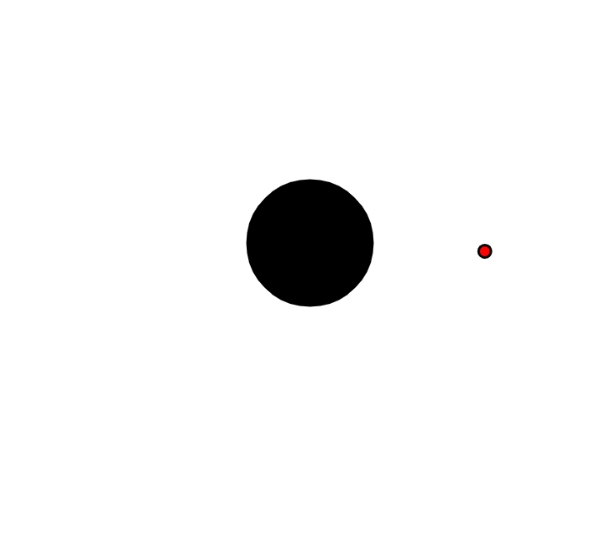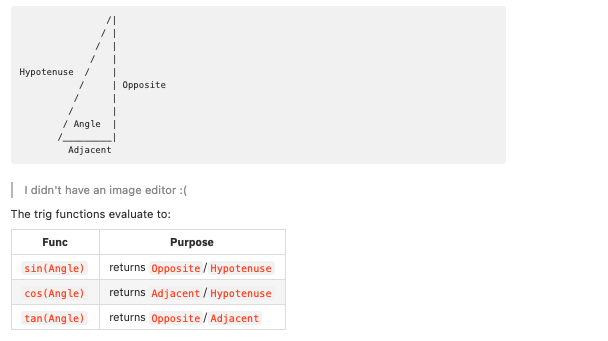Learn to Code via Tutorials on Repl.it!

← Back to all posts

# Shooting Tutorial

In many games, you would want to add something we like to call shooting -- that is, making projectiles go in a certain direction. It is actually very easy to accomplish this!! @BobTheTomatoPie hai

## Setup

First, we create an HTML repl with some minimum boilerplate. You can grab the fork here here. Long ridiculous name included!

## Creating a player

First, we must add a player. This is actually really easy to do. In `script.js`, we represent the player as:

``````// function draw() {
//   background(255);
fill(0);
ellipse(width / 2, height / 2, 50, 50);
// }``````

Note: The comments should not be added in the program, they are just to tell you where you should place the code!

## Creating the projectile class

The second thing we must do is to add projectiles (or bullets). The easiest way to represent this is with a class, so that is what we will do.

In the `projectile.js` file, add:

``````class Projectile {
constructor(x, y, ang) {
this.x = x;
this.y = y;
this.ang = ang;
}

draw() {
}
}``````

Note: It's always good to be organized!

our projectile class will take in the x position, the y position, and an angle which it will go at. We will next implement drawing the projectile.

``````  // draw() {
fill(0);
ellipse(this.x, this.y, 5, 5);
// }``````

While we are at it, let's also add an array that will contain our projectiles:

``````// class Projectile {
//   ...
// }

let projectiles = [];``````

## Shooting projectiles

Alright. Now that we have a class that abstracts a bullet, lets add the ability to shoot. In `script.js`, add:

``````// function draw() {
//  ...
// }

function mouseClicked() {
projectiles.push(new Projectile(width / 2, height / 2, ));
}``````

Now, the only argument we still need is the angle. How do we calculate the angle? Well, we know what our two points are, `(width / 2, height / 2)` and `(mouseX, mouseY)`. Calculating angles sounds pretty trigonometric to me, so we introduce the `atan2()`!

In trigonometry, we have three functions. They take in an angle, and return a ratio. Given a triangle like this:

``````                /|
/ |
/  |
/   |
Hypotenuse  /    |
/     | Opposite
/      |
/       |
/ Angle  |
/_________|
Adjacent``````

I didn't have an image editor :(

The trig functions evaluate to:

FuncPurpose
`sin(Angle)`returns `Opposite`/`Hypotenuse`
`cos(Angle)`returns `Adjacent`/`Hypotenuse`
`tan(Angle)`returns `Opposite`/`Adjacent`

Note: When calculating angles, which is an ambient context, the Hypotenuse is 1.

Now, because we have the side lengths of `Opposite` and `Adjacent` (remember those points?), we just need to use an inverse function to get back our angle! We use `atan2` because those are the sides we have. Now, if you are mathematically inclined, `atan2` actually isn't a function, `atan` is. The only thing is, `atan` has been used so much for things like our case that programmers decided to ease things up for us, by making the `atan2()` function. Here is what it takes in as the arguments:

``atan2(opposite, adjacent);``

So in our case, we have:

``atan2(mouseY - height / 2, mouseX - width / 2);``

All together now:

``````// function mouseClicked() {
projectiles.push(new Projectile(width / 2, height / 2, atan2(mouseY - height / 2, mouseX - width / 2)));
// }``````

## Drawing Projectiles

When you click on the screen, nothing happens! Why? We forgot to draw the projectiles! Let's draw them:

``````  // fill(0);
// ellipse(width / 2, height / 2, 50, 50);
//
projectiles.forEach(projectile => {
projectile.draw();
});``````

Next, we draw the projectiles. In `projectile.js`:

``````  // draw() {
fill(255, 0, 0);
ellipse(this.x, this.y, 5, 5);
// }``````

Note: This code is guaranteed to run after the page has loaded, and thus the p5.js variables are guaranteed to be defined.

Remember to always check if the page has loaded!

## Moving Projectiles

Fun fact: it still doesn't work!
The reason is that we still haven't actually added any code to move the projectiles.

Remember that triangle? Now that we have the angle, we want to calculate how much up and how much right we want to go. This sounds like a job for `sin()` and `cos()`.

``````  //   fill(255, 0, 0);
//   ellipse(this.x, this.y, 5, 5);

this.x += cos(this.ang);
this.y += sin(this.ang);``````

Note: If you read previous notes you would understand why this works.

Awesome! The only problem is that it is slightly slow, so let's speed it up.

``````    this.x += 5 * cos(this.ang);
this.y += 5 * sin(this.ang);``````

## Trying it out

When we click on the canvas to a certain spot, it works perfectly!You can check out the working repl here.

## Challenges

• Now that you know how to calculate the angle of the player and the cursor, add a gun!
• Add the ability to move the player.
• I recommend making player a class as well.

# Closing

I hoped you enjoyed this tutorial and you learned a lot! You can learn more about the library used, p5.js, and you can learn more math on Khan Academy.

If you liked this tutorial check out my game to see a reala world example (or any other shooter game for that case lol)!

##### Comments
hotnewtop
FranklinStopar (17)

...i got 20...lol put me on the scoreboard!

JBloves27 (1887)

Nice job!

At first, I thought it was a tutorial on how to kill things XD

Bookie0 (6294)

Shooting Tutorial

This just sounds wrong..

HEY! I'm learning that rn lol!

A way to remember this:

SOH CAH TOA
Sinus: Opposite / Hypothenuse
Cosinus: Adjacent / Hypothenuse
Tangente: Opposite / Adjacent

Or in french:

"CAHSOH-TOA"

which when you pronounce it makes "Casse-toi" meaning "Go away" (in a kinda vulgar way) xDDDDCoder100 (18207)

but this isn't a trig tutorial, it's just to tell you what is going on xd @Bookie0

FranklinStopar (17)

@Bookie0 its SoaCahToa not CahSoaToa....hahah

Bookie0 (6294)

@FranklinStopar no I made a typo it's SohCahToa

FranklinStopar (17)

@Bookie0 haha i was just messing with you i really dont care about the typo..

RhinoRunner (866)

I like the math!
JS is poop

Bunnytoes (157)

can you make a c# tutorial

cannonthepom123 (18)

could you do just a p5.js tutorial.

LingWu1 (94)

I got a high score of 91. Wow, that was quite stressful.